Home | | Structural Dynamics and Earthquake Engineering | Response of structures to periodic dynamic loadings

## Chapter: Civil : Structural dynamics of earthquake engineering

Abstract: In many real problems, it is found that exciting forces vary with time in a non-harmonic fashion that may be periodic or non-periodic. When the function defined over a period repeats indefinitely, it is known as a periodic function. In this page, we apply the Fourier series to determine the response of the system to periodic forces.

Abstract: In many real problems, it is found that exciting forces vary with time in a non-harmonic fashion that may be periodic or non-periodic. When the function defined over a period repeats indefinitely, it is known as a periodic function. In this page, we apply the Fourier series to determine the response of the system to periodic forces.

Key words: periodic function, Fourier series, odd function, even function, frequency domain, spectrum, Gibbs phenomenon.

Introduction

We have seen in Chapter 4 that harmonic excitation occurs in power installations equipped with rotating and reciprocating machinery. The solution to harmonic excitation is simple and straightforward since a closed form solution is always available. In many realistic situations, the exciting forces vary with time in a non-harmonic fashion that may be periodic or non-periodic. A periodic function is one in which the portion defined over a period T0 repeats itself indefinitely, (see Fig. 5.1). Propeller force on a ship, wind loading induced by vortex shedding on tall slender structures are the examples of periodic forces, whereas, earthquake ground motion has no resemblance to periodic function.

This chapter presents the application of Fourier series to determine the response of a system to periodic forces in the frequency domain, an alternative approach to the usual analysis of time domain. To make practical use of Fourier method, it is necessary to replace the integration with finite sums.Fourier analysis

Consider an undamped single-degree-of-freedom (SDOF) system subjected to force F(t)

The governing equation is written as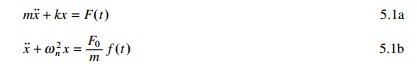Let the forcing function be represented as shown in Fig. 5.2. The duration of one pulse or period T0 = 4s.

F(t) = 10 t <2 s

F(t) = ├É10 2 < t < 4 5.2

Fourier has shown that a periodic function can be expressed as a sum of infinite number of sine and cosine terms and such a sum is known as a Fourier series.t1 in Eq. 5.5 can take any value of time, but is usually ├É T/2 or zero. Note that Fourier coefficients a1, an, bn may be expedited if the forcing function can be recognized as being odd or even. If the forcing function is anti-symmetric about the origin (y-axis) then it is an odd function. For example, the given function shown in Fig. 5.2 is an odd function becauseFor an odd function, the Fourier coefficients a0, a1,├ē, an = 0

The forcing function is even ifFor an even function, the Fourier coefficients b0, b1,├ē, bn = 0

Clearly then an odd function can be represented by the Fourier sine series and an even function by the Fourier cosine series. If, however, the function is neither odd nor even, then the full Fourier series must be employed. The Fourier coefficients can very easily be evaluated using the MATHEMATICA package as shown by the following examples.

Example 5.1

Determine the Fourier series expression for the square wave, periodic function as shown in Fig. 5.2. Plot the Fourier representation for the first four non-zero harmonic components. (Assume F0 = 10)

Solution

Since the forcing function is an odd function, the Fourier coefficients a0, a1,├ē, an = 0. Thus the forcing function is represented by the Fourier sine series as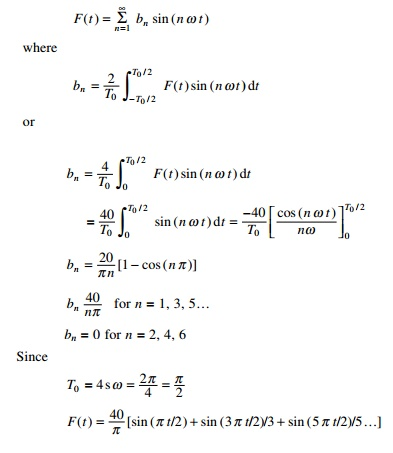The forcing function is represented in Fig. 5.3 in terms of 1, 2, 3 and 4 non-zero terms of Fourier series.

Example 5.2

Determine the Fourier series expression for square wave periodic forcing function as shown in Fig. 5.4. Plot the Fourier representation of the first four non-zero components.Solution

Since F(t) is an even function, b1, b2, bn are zero. Thus ├'a├Ģ coefficients are represented by Fourier cosine series asIn Fig. 5.5 the forcing function is represented in terms of 1, 2, 3, 4 non-zero terms of cosine series.

The MATHEMATICA program is used to find the Fourier coefficients.

Example 5.3

Determine the Fourier series expansion for a square wave periodic forcing function as shown in Fig. 5.6. Plot the Fourier representation of the first four non-zero componentsSolution

Since F(t) is neither odd nor even function, this should contain both sine and cosine terms. In Fig. 5.6 the forcing function is represented in terms of 1, 2, 3 and 4 non-zero terms of Fourier series.

Response to periodic excitation

Next, consider the response of a viscously damped SDOF system to periodic non-harmonic excitation of period T0. The differential equation of motion of steady state response is given byin which Fourier series expansion was used to represent F(t). The transient response will decay with time and is hence neglected. To obtain the particular solution for the steady state response, it is noted that differential equation is linear, and therefore principle of superposition is applicable. Hence the steady state response is merely the sum of the individual particular solutions for all harmonic terms representing F(t). Hence we obtainin which Žłn is the phase angle for the steady state response.

Example 5.4

For the harmonic periodic forcing function shown in Fig. 5.5 determine the steady state response for a viscously under-damped SDOF system.Example 5.5

The building frame shown in Fig. 5.7 is constructed of rigid girders and flexible columns. The frame supports a uniformly distributed load of weight of 130kN and is subjected at its girder level to the periodic force described in Fig. 5.4. Evaluate the steady state response of the structure and plot displacement vs. time assuming F0 = 80kN, period of exciting force is 2 s, E = 200GPa, damping factor = 0.1, height of end columns is 4m, the height of centre column is 6m and the span of the beams is 6m. The values of I for end columns and centre column are 2.6 ├- 108 and 3.254 ├- 108mm4 respectively.Example 5.6

Consider a system loaded as shown in Fig. 5.9. Find the steady state response.in which Ž- = 2ŽĆ/T0.

Using the MATHEMATICA program, the displacement response is obtained for ╬▓ = ŽĆ/4, T0 = 4s Žü = 0.25 as shown in Fig. 5.10.

Frequency domain analysis

The dynamic response of the complex systems can be examined by two approaches: time domain solution and the frequency domain solution. Up to now, all solutions have been in time domain and the equations are solved by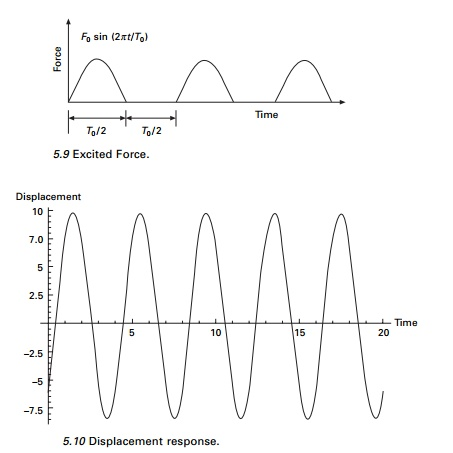integrating with time. In frequency domain technique, the amplitude coefficients in the Fourier series solution corresponding to each frequency are determined. For this we use discrete Fourier transform (DFT) or fast Fourier transform (FFT) methods.

Alternative form of Fourier series

The arbitrary periodic forcing function can be represented in the form of Fourier series (see Eq. 5.3) asin which a0, a1├ē an, b1, b2, ├ē bn are real constants, t is the time and Žē is the forcing frequency given by Žē = 2ŽĆ/T0 where T0 is the period of the forcing function. If the period of the forcing function is not known or if the forcing function does not have period for this case Žē = 2ŽĆ/TD where TD is the total time duration of the forcing function.

Equation 5.11 may also be written as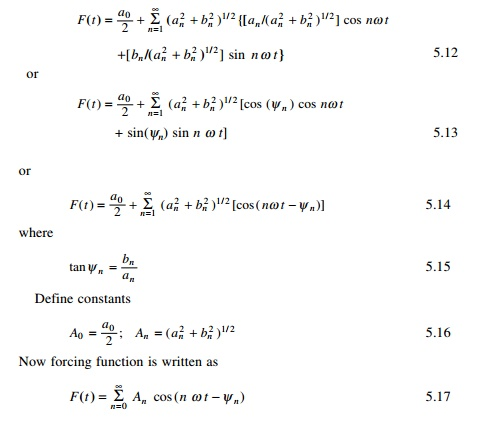in which Žł0 = 0. Hence forcing function is now written in terms of positive real amplitude and phase shift. This method replaces the coefficients of an, bn by An, Žłn. There is still the same number of coefficients. The plots of An and Žłn are called amplitude spectrum and phase spectrum respectively. They are discrete and occur at Fourier frequencies nŽē = (2ŽĆ n)/TD.

Example 5.7

A periodic load function of three frequency components is given by

F(t) = 10 cos (Žē t - 0.5) + 7 cos (2Žē t - 0.2) + 2 cos (3 Žē t - 0.1)

in which Žē = 2ŽĆ/TD and TD = 5s. Plot the periodic function and the amplitude and phase spectrum.

Solution

The equation for the coefficients are given asexcept that we use TD as fundamental period. Evaluating the integrals in the above equations yields the values given in Table 5.1.Program 5.3: MATLAB program to evaluate amplitudes and phase angles

clc; close all;

for i=1:200 t(i)=(i-1)/40;

p(i)=10*cos(2*pi*t(i)/5├É0.5)+7.0*cos(4*pi*t(i)/5├É0.2)+2.0*cos(6.0*pi*t(i)/

5├É0.1) end figure(1) plot(t,p) xlabel(' t');

ylabel('p(t)');

title(' time series plot'); figure(2)

a=fft(p);

plot(abs(a)); title(' amplitude') for i=1:200

b(i)=real(a(i));

c(i)=imag(a(i)); d(i)=atan(-c(i)/b(i)); e(i)=sqrt(b(i)^2+c(i)^2);

end

print{' real part',\n); b

print(' imaginary part',\n); c

print(' phase angle',\n); d

print(' amplitude',\n)' e

figure(3)

plot(d); title(' phase') figure(4);

plot(t,b,t,c,'*');

title(' real and imaginary part(*)')

The forcing function F(t) examined in Example 5.6 is rather a simple function, although, from the plot presented in Fig. 5.11a it is not readilyapparent that this is a simple function. However the amplitude and phase spectrum parts shown in Fig. 5.11b and 5.11c reveal clearly three cosines and their phases. As functions become more complex with many frequency components, plots of amplitude spectra become even more important in understanding the structure of the functions.

Example 5.8

A simple periodic load is characterized by the following deterministic amplitude coefficientsExpression of forcing function using complex variable approach

The forcing function is written asThe exponential form is more convenient to use than the real form. To illustrate the fact, consider SDOF system subjected to harmonic forcing. The equation of motion is given by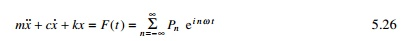The steady state solution of Eq. 5.26 will respond to same frequencies making up the forcing function.Writing in this way, the response at each frequency is simply a function of the forcing transfer function. For a given structure H depends only on frequency nŽē. This is termed the frequency domain solution. Consider ╬▓ as the frequency ratio and Žü as the damping factor. The function Hn is written as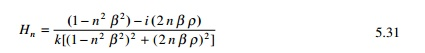The phase is now defined as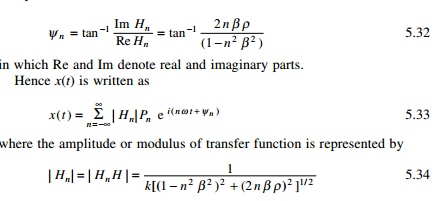where Hn* is the complex conjugate of Pn and Žån is the phase. It is to be noted that forcing function Pn can be complex. Expressing Pn as a modulus and phase we getSince the spectral coefficients are even, this implies that a spectrum plot we can consider either all frequencies between ├ÉŌł× and Ōł× or just frequencies from 0 to Ōł× and double the heights. The former is called a double-sided spectrum and the latter is called a single-sided spectrum. Both spectra are reasonable representations and both are used in practice.

Discrete Fourier transform (DFT) and fast Fourier transform (FFT)

Although Fourier integral technique discussed in previous sections provide a means for determining the transient response of a system, numerical integral of the Fourier integral became a practical reality only with the publication of Cooley-Tukey algorithm for the FFT in 1965. Since that date, FFT has revolutionized many areas of technology such as the areas of measurements and instrumentation.

Two steps are involved in the numerical evaluation of Fourier transforms. DFTs correspond to the equationwhere N is the number of points in the time series approximation of F(t). We can writeThe total number of discrete time values and frequency values are same. The N term appearing before the summation in Eq. 5.40 is not unique. In

Root o

DFT it can be used as 1/N in Eqs. 5.40 and 5.41 or   N  Root in both.

Example 5.10

Determine the DFT of the following function defined by

f (n Ōłåt) = 6 + 14 sin (nŽĆ/2) ├ē n = 0, 1, 2,├ē, 7

Solution

Before developing a solution using the DFT, it is useful to first examine the time series. The discrete trigonometric Fourier series is expressed asIn this example N = 8 and all coefficients are zero except a0 = 12; b2 = 14. For this problem the solution could be determined by inspection. With use of the Euler formulae, the time series is written asThe transform of the series is given by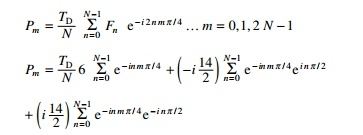These coefficients may be evaluated using the MATHEMATICA package and given byThese are the same coefficients that were determined by inspection of equation defining Pn, Pm = Pm+n or P├É2 = P6.

Gibbs phenomenon

The Fourier series approximation of a square wave has been plotted in Fig. 5.34. The approximation is generally quite good as shown in the figure. However, an inaccuracy exists at the corners of the wave. Sines and cosines are smooth, continuous functions and therefore are best suited to approximately other smooth and continuous functions. However, jumps or discontinuities exist in them and the approximation is poor. For the square wave a discontinuity exists at t/T0 = 0.5. At this location, the square wave has two values +1 and ├É1. When the function has jumps or double values, a Fourier series passes through the mean of the two points as shown in Fig. 5.34, which in our case is zero. It is also to be noted that t/T0 = 0.5 the square wave is vertical. The fourier series tends to overshoot at the corners. This is called the Gibbs phenomenon. This does not disappear even if large number of terms are used

in the series. It is concluded that the Gibbs phenomenon is local and the contribution to total energy is minimal.

Summary

Fourier integrals can be evaluated numerically. There are a number of software packages available to do this, such as MATHEMATICA and MATLAB. DFT has a finite number of frequencies and Fourier integrals have continuous frequencies in the interval ├ÉŌł× < f < Ōł× and high frequency terms are lost. The other way of doing the Fourier integral is to use polynomial interpolation functions which can be integrated analytically over most of the data.

Study Material, Lecturing Notes, Assignment, Reference, Wiki description explanation, brief detail
Civil : Structural dynamics of earthquake engineering : Response of structures to periodic dynamic loadings |